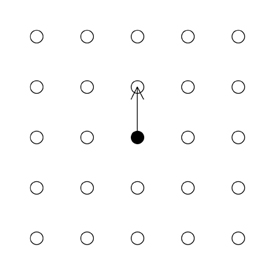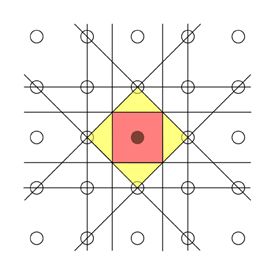# 8.3: Brillouin Zone construction

$$\newcommand{\vecs}{\overset { \rightharpoonup} {\mathbf{#1}} }$$ $$\newcommand{\vecd}{\overset{-\!-\!\rightharpoonup}{\vphantom{a}\smash {#1}}}$$$$\newcommand{\id}{\mathrm{id}}$$ $$\newcommand{\Span}{\mathrm{span}}$$ $$\newcommand{\kernel}{\mathrm{null}\,}$$ $$\newcommand{\range}{\mathrm{range}\,}$$ $$\newcommand{\RealPart}{\mathrm{Re}}$$ $$\newcommand{\ImaginaryPart}{\mathrm{Im}}$$ $$\newcommand{\Argument}{\mathrm{Arg}}$$ $$\newcommand{\norm}{\| #1 \|}$$ $$\newcommand{\inner}{\langle #1, #2 \rangle}$$ $$\newcommand{\Span}{\mathrm{span}}$$ $$\newcommand{\id}{\mathrm{id}}$$ $$\newcommand{\Span}{\mathrm{span}}$$ $$\newcommand{\kernel}{\mathrm{null}\,}$$ $$\newcommand{\range}{\mathrm{range}\,}$$ $$\newcommand{\RealPart}{\mathrm{Re}}$$ $$\newcommand{\ImaginaryPart}{\mathrm{Im}}$$ $$\newcommand{\Argument}{\mathrm{Arg}}$$ $$\newcommand{\norm}{\| #1 \|}$$ $$\newcommand{\inner}{\langle #1, #2 \rangle}$$ $$\newcommand{\Span}{\mathrm{span}}$$

The reciprocal lattice basis vectors span a vector space that is commonly referred to as reciprocal space, or often in the context of quantum mechanics, k space. This section covers the construction of Brillouin zones in two dimensions.

The first step is to use the real space lattice vectors to find the reciprocal lattice vectors and construct the reciprocal lattice. One of the points in the reciprocal lattice is then designated to be the origin. When constructing Brillouin zones, they are always centred on a reciprocal lattice point, but it is important to keep in mind that there is nothing special about this point as each reciprocal lattice point is equivalent due to translation symmetry.

Draw a line connecting this origin point to one of its nearest neighbours. This line is a reciprocal lattice vector as it connects two points in the reciprocal lattice.The second Brillouin Zone is the region of reciprocal space in which a point has one Bragg Plane between it and the origin. This area is shaded yellow in the picture below. Note that the areas of the first and second Brillouin Zones are the same.The construction can quite rapidly become complicated as you move beyond the first few zones, and it is important to be systematic so as to avoid missing out important Bragg Planes.

Illustration that follows this process to show the first six Brillouin Zones for the 2-D square reciprocal lattice.Illustration showing the first six zones for a 2-D hexagonal reciprocal lattice.